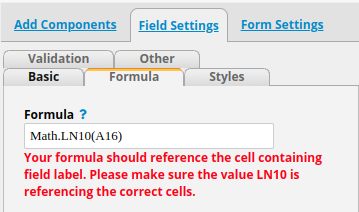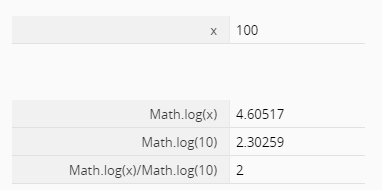# Asked to ensure LN10 is referencing the correct cells while Math.LN10 was used

Error Message:
Your formula should reference the cell containing field label. Please make sure the value LN10 is referencing the correct cells.

My thought:
Looks like LN10 was recognized as a reference to a cell rather than formula.

Below is how I apply the formulaAre there hidden rules to apply formula under Math.*?
Please share with me how you apply formula under Math.*, especially LN10.

Thanks,
Jonah

UPDATE
I just realize I could use `Math.log(A16)` to get the natural log of `A16`. Therefore, my issue is solved for now. But I still think that this page is not easy to understand.

I also tried to apply formula below, believing it will return log10 of the value 100. However, this formula return neither error nor any value.

``````Math.LN(100, 10)
``````

Hi,
based on the page.
Math.LN10 itself will return a value for you.
However, when I tried this in the formula, it didn’t work.

So I tried using Javascript to see if it’s able to calculate it. And it works.
However, I still wanna try using the existing Ragic formula to see if there’s anyway to get the same value.
Turns out that Math.log(x) (for natural logarithm) does work. However, since that Math.LN10 doesn’t work properly when I use it in the formula and I have long forgotten about logarithm math, so I enlisted ChatGPT for some enlightment.
And I was able to find that you can get Log10 value without using Log10 function specifically.

You can try using this formula:
Math.log(x)/Math.log(10)

Note: be aware of the caption in the formula. I accidentally used Math.Log(x) with a Cap L and it doen’t work.1 Like

You might already notice that I was taking `Math.LN10` as a method rather than a `constant`. I think this is the beginning of my mistake.